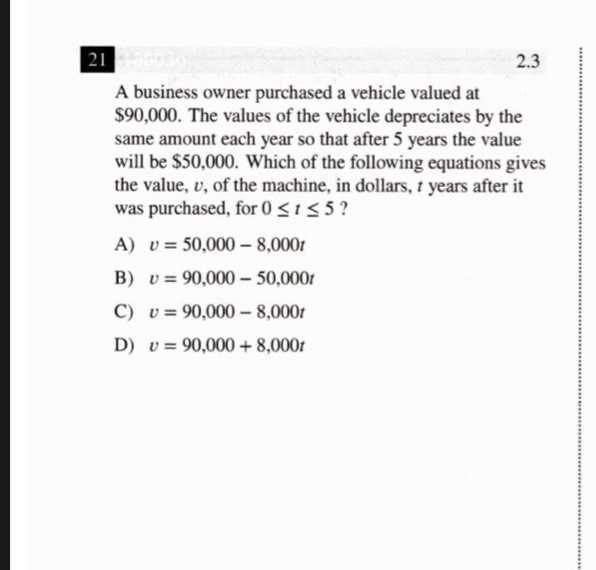### ¿Todavía tienes preguntas de matemáticas?

Pregunte a nuestros tutores expertos
Algebra
PreguntaA business owner purchased a vehicle valued at $$\ 90,000$$ . The values of the vehicle depreciates by the same amount each year so that after $$5$$ years the value will be $$\ 50,000$$ . Which of the following equations gives the value, $$v$$ , of the machine, in dollars, $$t$$ years after it was purchased, for $$0 \leq t \leq 5$$ ?

A) $$v = 50,000 - 8,000 t$$

B) $$v = 90,000 - 50,000 t$$

C) $$v = 90,000 - 8,000 t$$

D) $$v = 90,000 + 8,000 t$$